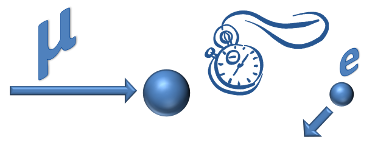In these exercises, students will study events in which a muon coming to rest inside the MINERvA detector subsequently undergoes a radioactive decay in mere microseconds.Students learn how to identify radioactive decay events and get a feel for the random nature of the decay process. Later, a more quantitative analysis can measure the half-life of the muon. Students also examine the energies of the electrons to gain insight into the decay mechanism.

The sample event above shows a muon beta decay. The second "time slice" in this event contains a muon skidding to a halt in the material of the MINERvA detector. The third time slice reveals a 35 Mega-electron-Volt electron emmitted by the muon when it decays radioactively 2.45 microseconds after it stopped in the detector.

This exercise provides a library of these decay events for your students to analyze.

### Essential Questions

• What is the distribution of the random decay times? Why is it an exponential distribution?

### Activities and Resources

• Small group and large group analysis of radioactive decays of muons using Arachne and Excel worksheets

### Essential Questions

• How is the decay of a muon like the radioactive decay I learned about in chemistry?
• In what way is radioactive decay a random process?

### Activities and Resources

• Explore the different types of decay and the processes at the Particle Adventure website:
• Learning how to identify muon decays in MINERvA with Arachne:
• Use the Arachne display to identify muon decays and the resulting "Michel" electron
• Arachne basics for muon decay (Link into teacher's guide)
• Event samples: give each group of students one of the links below:
 Group A Group K Group B Group L Group C Group M Group D Group N Group E Group O Group F Group P Group G Group Q Group H Group R Group I Group S Group J Group T
• For teacher's reference, some of the events in the list of links above have solutions as shown in the spreadsheet below. Events are labelled by MV|run|subrun|event, which is visible in Arachne (in the 'Slice Info' window).